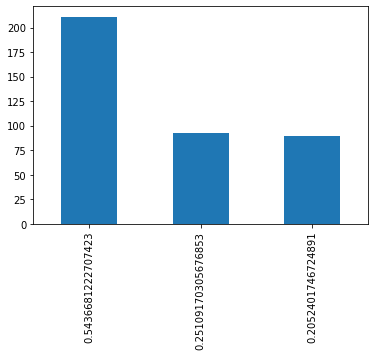# CountFrequencyEncoder¶

The CountFrequencyEncoder() replaces categories by the count of observations per category or by the percentage of observations per category.
For example in the variable colour, if 10 observations are blue, blue will be replaced by 10. Alternatively, if 10% of the observations are blue, blue will be replaced by 0.1.

In :
import pandas as pd
import numpy as np
import matplotlib.pyplot as plt

from sklearn.model_selection import train_test_split
from feature_engine.encoding import CountFrequencyEncoder

In :
# Load titanic dataset from OpenML

data = data.replace('?', np.nan)
data['cabin'] = data['cabin'].astype(str).str
data['pclass'] = data['pclass'].astype('O')
data['age'] = data['age'].astype('float')
data['fare'] = data['fare'].astype('float')
data['embarked'].fillna('C', inplace=True)
data.drop(labels=['boat', 'body', 'home.dest'], axis=1, inplace=True)
return data

In :
data = load_titanic()

Out:
pclass survived name sex age sibsp parch ticket fare cabin embarked
0 1 1 Allen, Miss. Elisabeth Walton female 29.0000 0 0 24160 211.3375 B S
1 1 1 Allison, Master. Hudson Trevor male 0.9167 1 2 113781 151.5500 C S
2 1 0 Allison, Miss. Helen Loraine female 2.0000 1 2 113781 151.5500 C S
3 1 0 Allison, Mr. Hudson Joshua Creighton male 30.0000 1 2 113781 151.5500 C S
4 1 0 Allison, Mrs. Hudson J C (Bessie Waldo Daniels) female 25.0000 1 2 113781 151.5500 C S
In :
X = data.drop(['survived', 'name', 'ticket'], axis=1)
y = data.survived

In :
# we will encode the below variables, they have no missing values
X[['cabin', 'pclass', 'embarked']].isnull().sum()

Out:
cabin       0
pclass      0
embarked    0
dtype: int64
In :
''' Make sure that the variables are type (object).
if not, cast it as object , otherwise the transformer will either send an error (if we pass it as argument)
or not pick it up (if we leave variables=None). '''

X[['cabin', 'pclass', 'embarked']].dtypes

Out:
cabin       object
pclass      object
embarked    object
dtype: object
In :
# let's separate into training and testing set
X_train, X_test, y_train, y_test = train_test_split(X, y, test_size=0.3, random_state=0)

X_train.shape, X_test.shape

Out:
((916, 8), (393, 8))

The CountFrequencyEncoder(), replaces the categories by the count or frequency of the observations in the train set for that category.

If we select "count" in the encoding_method, then for the variable colour, if there are 10 observations in the train set that show colour blue, blue will be replaced by 10.

Alternatively, if we select "frequency" in the encoding_method, if 10% of the observations in the train set show blue colour, then blue will be replaced by 0.1.

### Frequency¶

Labels are replaced by the percentage of the observations that show that label in the train set.

In :
'''
Parameters
----------

encoding_method : str, default='count'
Desired method of encoding.

'count': number of observations per category

'frequency': percentage of observations per category

variables : list
The list of categorical variables that will be encoded. If None, the
encoder will find and transform all object type variables.
'''
count_encoder = CountFrequencyEncoder(encoding_method='frequency',
variables=['cabin', 'pclass', 'embarked'])

count_encoder.fit(X_train)

Out:
CountFrequencyEncoder(encoding_method='frequency',
variables=['cabin', 'pclass', 'embarked'])
In :
# we can explore the encoder_dict_ to find out the category replacements.
count_encoder.encoder_dict_

Out:
{'cabin': {'n': 0.7663755458515283,
'C': 0.07751091703056769,
'B': 0.04585152838427948,
'D': 0.034934497816593885,
'E': 0.034934497816593885,
'A': 0.018558951965065504,
'F': 0.016375545851528384,
'G': 0.004366812227074236,
'T': 0.001091703056768559},
'pclass': {3: 0.5436681222707423,
1: 0.25109170305676853,
2: 0.2052401746724891},
'embarked': {'S': 0.7117903930131004,
'C': 0.19759825327510916,
'Q': 0.0906113537117904}}
In :
# transform the data: see the change in the head view
train_t = count_encoder.transform(X_train)
test_t = count_encoder.transform(X_test)

Out:
pclass sex age sibsp parch fare cabin embarked
1139 0.543668 male 38.0 0 0 7.8958 0.766376 0.71179
533 0.205240 female 21.0 0 1 21.0000 0.766376 0.71179
459 0.205240 male 42.0 1 0 27.0000 0.766376 0.71179
1150 0.543668 male NaN 0 0 14.5000 0.766376 0.71179
393 0.205240 male 25.0 0 0 31.5000 0.766376 0.71179
In :
test_t['pclass'].value_counts().plot.bar()
plt.show()In :
test_orig = count_encoder.inverse_transform(test_t)

Out:
pclass sex age sibsp parch fare cabin embarked
1139 3 male 38.0 0 0 7.8958 n S
533 2 female 21.0 0 1 21.0000 n S
459 2 male 42.0 1 0 27.0000 n S
1150 3 male NaN 0 0 14.5000 n S
393 2 male 25.0 0 0 31.5000 n S

### Count¶

Labels are replaced by the number of the observations that show that label in the train set.

In :
# this time we encode only 1 variable

count_enc = CountFrequencyEncoder(encoding_method='count',
variables='cabin')

count_enc.fit(X_train)

Out:
CountFrequencyEncoder(variables=['cabin'])
In :
# we can find the mappings in the encoder_dict_ attribute.

count_enc.encoder_dict_

Out:
{'cabin': {'n': 702,
'C': 71,
'B': 42,
'D': 32,
'E': 32,
'A': 17,
'F': 15,
'G': 4,
'T': 1}}
In :
# transform the data: see the change in the head view for Cabin

train_t = count_enc.transform(X_train)
test_t = count_enc.transform(X_test)


Out:
pclass sex age sibsp parch fare cabin embarked
1139 3 male 38.0 0 0 7.8958 702 S
533 2 female 21.0 0 1 21.0000 702 S
459 2 male 42.0 1 0 27.0000 702 S
1150 3 male NaN 0 0 14.5000 702 S
393 2 male 25.0 0 0 31.5000 702 S

### Select categorical variables automatically¶

If we don't indicate which variables we want to encode, the encoder will find all categorical variables

In :
# this time we ommit the argument for variable
count_enc = CountFrequencyEncoder(encoding_method = 'count')

count_enc.fit(X_train)

Out:
CountFrequencyEncoder(variables=['pclass', 'sex', 'cabin', 'embarked'])
In :
# we can see that the encoder selected automatically all the categorical variables

count_enc.variables

Out:
['pclass', 'sex', 'cabin', 'embarked']
In :
# transform the data: see the change in the head view

train_t = count_enc.transform(X_train)
test_t = count_enc.transform(X_test)


Out:
pclass sex age sibsp parch fare cabin embarked
1139 498 581 38.0 0 0 7.8958 702 652
533 188 335 21.0 0 1 21.0000 702 652
459 188 581 42.0 1 0 27.0000 702 652
1150 498 581 NaN 0 0 14.5000 702 652
393 188 581 25.0 0 0 31.5000 702 652

### Note¶

if there are labels in the test set that were not present in the train set, the transformer will introduce NaN, and raise a warning.

In [ ]: